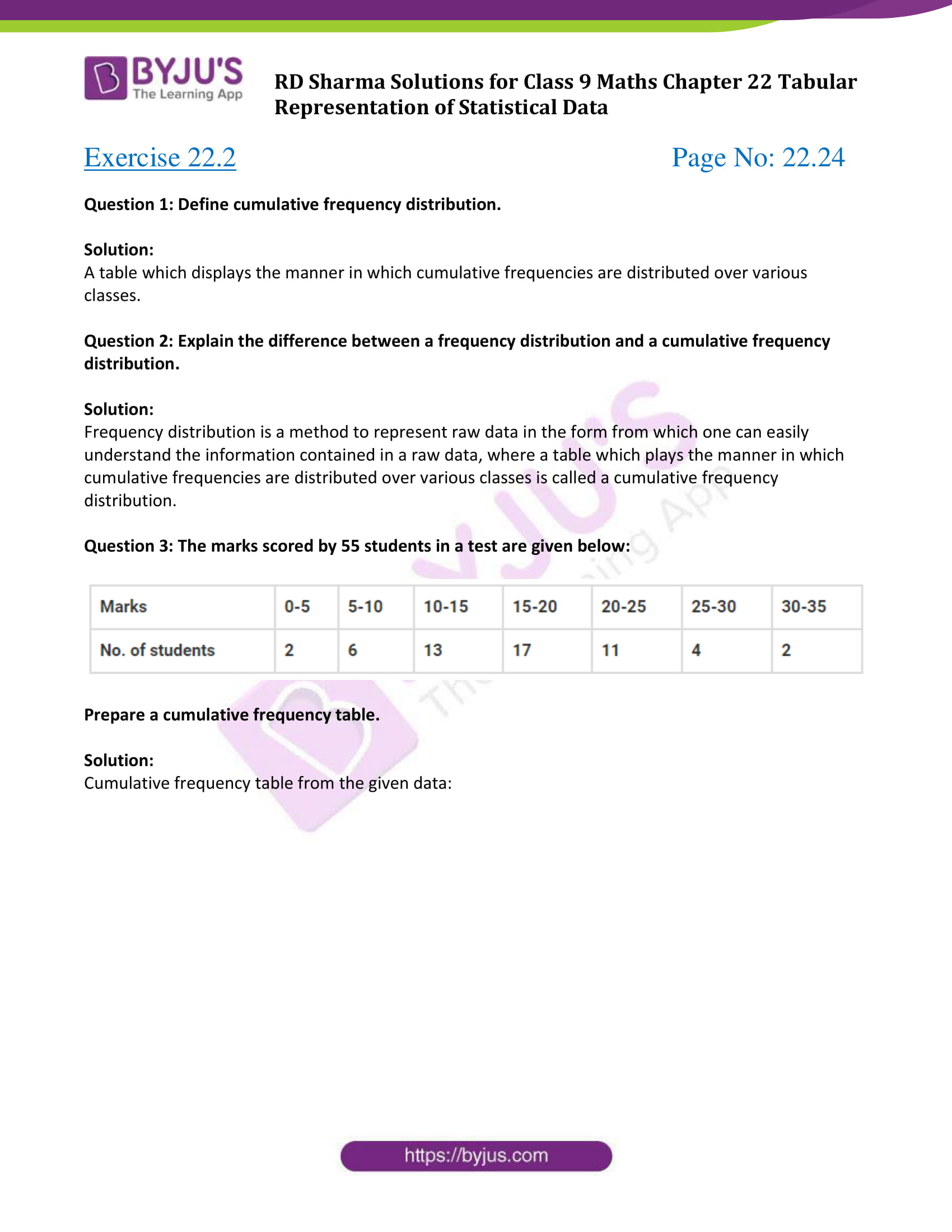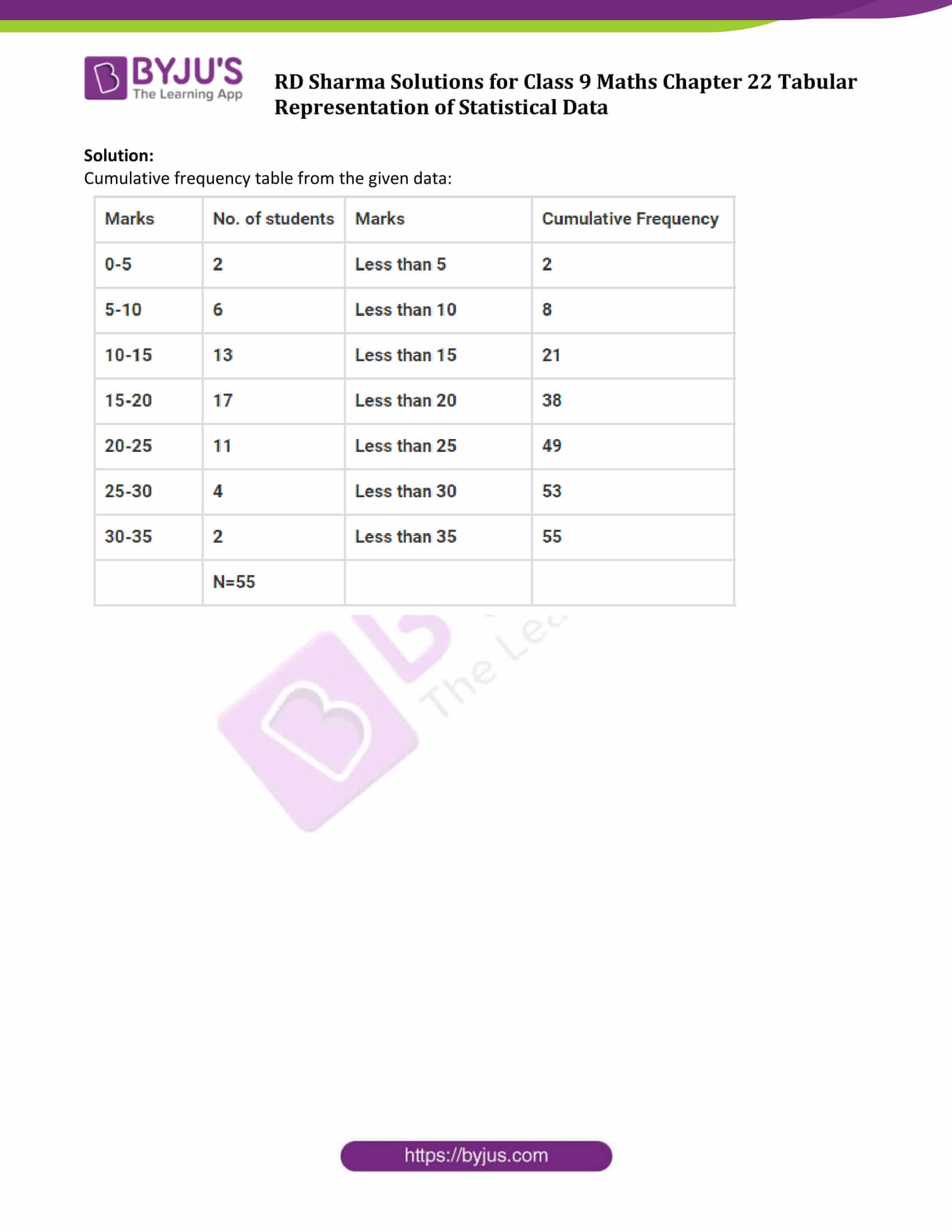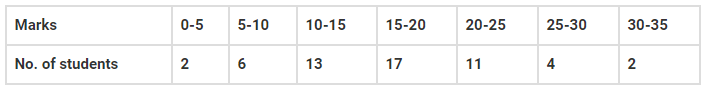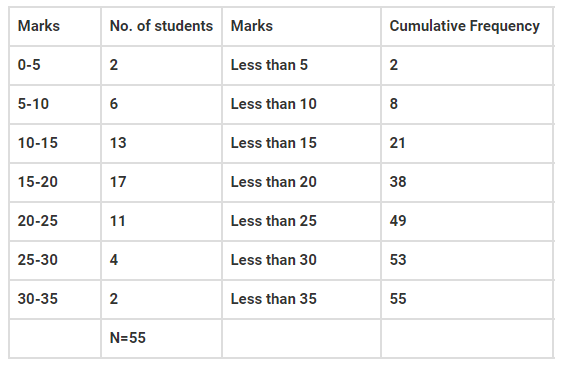# RD Sharma Solutions for Class 9 Maths Chapter 22 Tabular Representation of Statistical Data Exercise 22.2

Class 9 Chapter 22 – Tabular Representation of Statistical Data Exercise 22.2 solutions are provided here. These solutions for CBSE Class 9 are outlined keeping in mind the current CBSE syllabus, hence possessing a great chance of appearing and scoring good marks in the exams. In this section, students will learn about cumulative frequency distribution. Click on the link below to get your pdf for free now. RD Sharma Solution class 9 chapter 22 are outlined and solved by the experts at BYJU’S.

## Download PDF of RD Sharma Solutions for Class 9 Maths Chapter 22 Tabular Representation of Statistical Data Exercise 22.2### Access Answers to Maths RD Sharma Solutions for Class 9 Chapter 22 Tabular Representation of Statistical Data Exercise 22.2 Page number 22.24

Question 1: Define cumulative frequency distribution.

Solution:

A table which displays the manner in which cumulative frequencies are distributed over various classes.

Question 2: Explain the difference between a frequency distribution and a cumulative frequency distribution.

Solution:

Frequency distribution is a method to represent raw data in the form from which one can easily understand the information contained in a raw data, where a table which plays the manner in which cumulative frequencies are distributed over various classes is called a cumulative frequency distribution.

Question 3: The marks scored by 55 students in a test are given below:Prepare a cumulative frequency table.

Solution:

Cumulative frequency table from the given data:## RD Sharma Solutions for Class 9 Maths Chapter 22 Tabular Representation of Statistical Data Exercise 22.2

RD Sharma Solutions Class 9 Maths Chapter 22 Tabular Representation of Statistical Data Exercise 22.2 is based on the topic – Cumulative frequency distribution. In this exercise, students will learn how to construct cumulative frequency distribution table using given data.